# Curiousily

## Demand Prediction with LSTMs using TensorFlow 2 and Keras in Python

Deep Learning, Keras, TensorFlow, Time Series, Python3 min read

Share

TL;DR Learn how to predict demand using Multivariate Time Series Data. Build a Bidirectional LSTM Neural Network in Keras and TensorFlow 2 and use it to make predictions.

One of the most common applications of Time Series models is to predict future values. How the stock market is going to change? How much will 1 Bitcoin cost tomorrow? How much coffee are you going to sell next month?

Haven’t heard of LSTMs and Time Series? Read the previous part to learn the basics.

This guide will show you how to use Multivariate (many features) Time Series data to predict future demand. You’ll learn how to preprocess and scale the data. And you’re going to build a Bidirectional LSTM Neural Network to make the predictions.

Here are the steps you’ll take:

Run the complete notebook in your browser

The complete project on GitHub

# Data

Our data London bike sharing dataset is hosted on Kaggle. It is provided by Hristo Mavrodiev. Thanks!

A bicycle-sharing system, public bicycle scheme, or public bike share (PBS) scheme, is a service in which bicycles are made available for shared use to individuals on a short term basis for a price or free. - Wikipedia

Our goal is to predict the number of future bike shares given the historical data of London bike shares. Let’s download the data:

1!gdown --id 1nPw071R3tZi4zqVcmXA6kXVTe43Ex6K3 --output london_bike_sharing.csv

and load it into a Pandas data frame:

1df = pd.read_csv(2  "london_bike_sharing.csv",3  parse_dates=['timestamp'],4  index_col="timestamp"5)

Pandas is smart enough to parse the timestamp strings as DateTime objects. What do we have? We have 2 years of bike-sharing data, recorded at regular intervals (1 hour). And in terms of the number of rows:

1df.shape
1(17414, 9)

That might do. What features do we have?

• timestamp - timestamp field for grouping the data
• cnt - the count of a new bike shares
• t1 - real temperature in C
• t2 - temperature in C “feels like”
• hum - humidity in percentage
• wind_speed - wind speed in km/h
• weather_code - category of the weather
• is_holiday - boolean field - 1 holiday / 0 non holiday
• is_weekend - boolean field - 1 if the day is weekend
• season - category field meteorological seasons: 0-spring ; 1-summer; 2-fall; 3-winter.

How well can we predict future demand based on the data?

# Feature Engineering

We’ll do a little bit of engineering:

1df['hour'] = df.index.hour2df['day_of_month'] = df.index.day3df['day_of_week'] = df.index.dayofweek4df['month'] = df.index.month

All new features are based on the timestamp. Let’s dive deeper into the data.

# Exploration

Let’s start simple. Let’s have a look at the bike shares over time: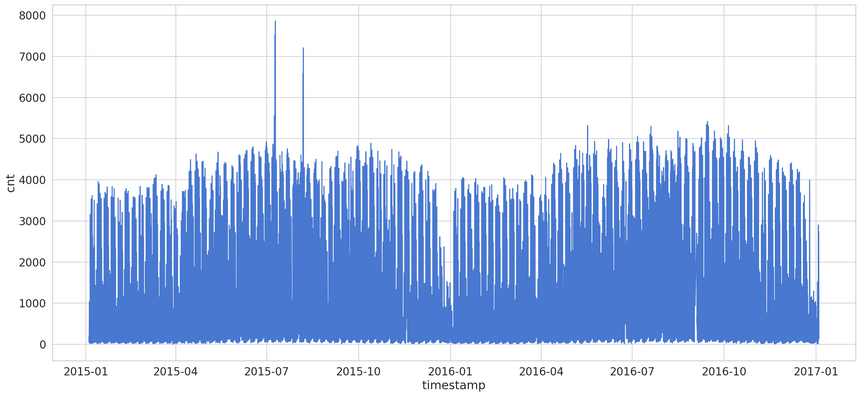That’s a bit too crowded. Let’s have a look at the same data on a monthly basis: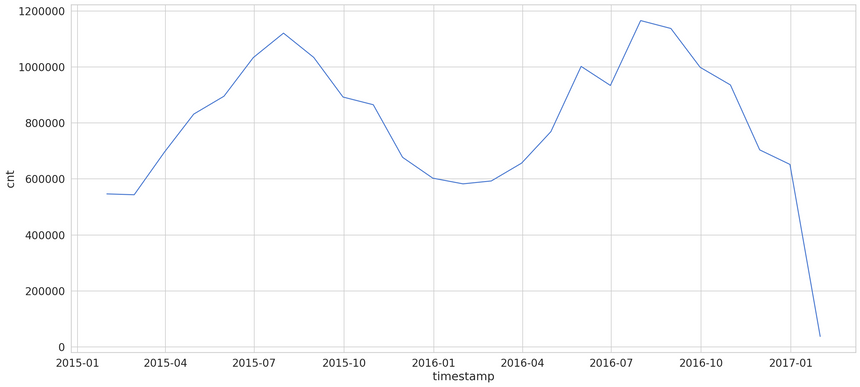Our data seems to have a strong seasonality component. Summer months are good for business.

How about the bike shares by the hour: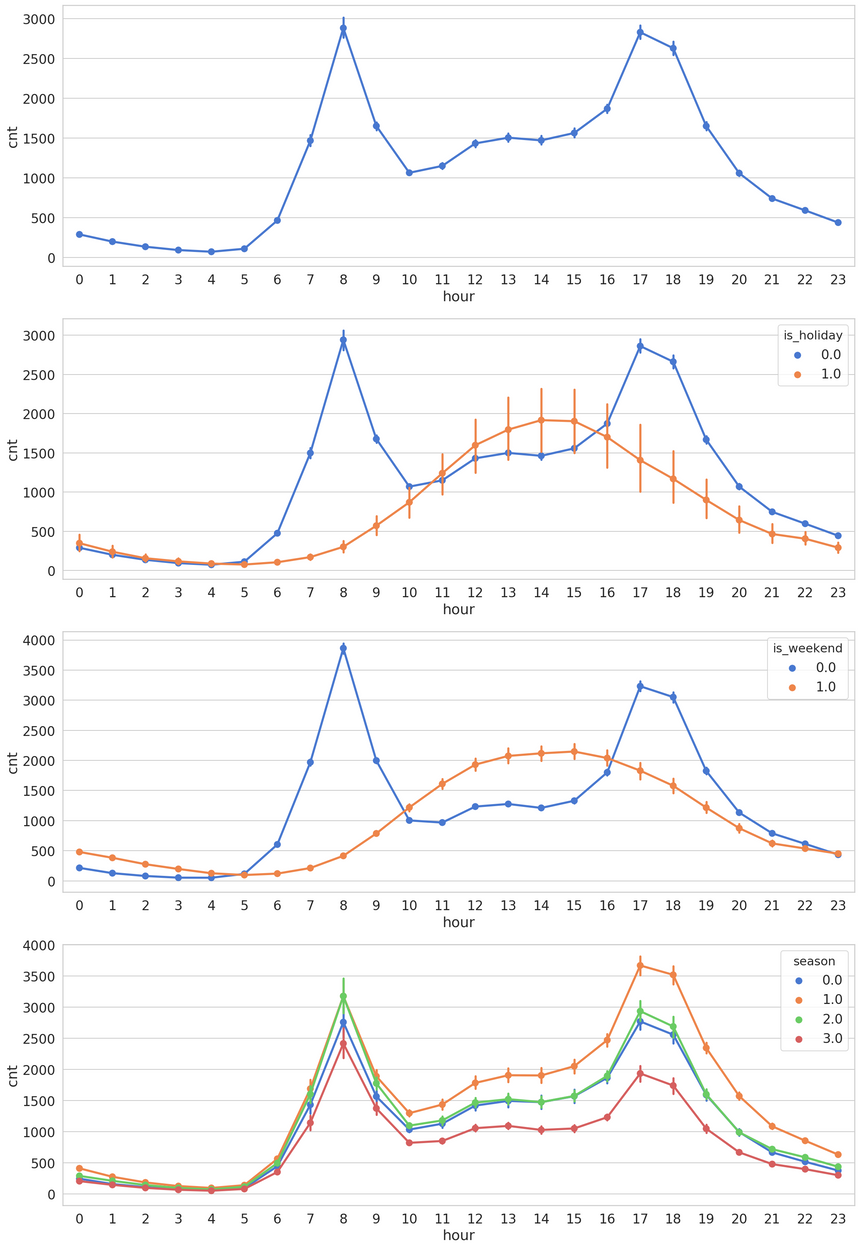The hours with most bike shares differ significantly based on a weekend or not days. Workdays contain two large spikes during the morning and late afternoon hours (people pretend to work in between). On weekends early to late afternoon hours seem to be the busiest.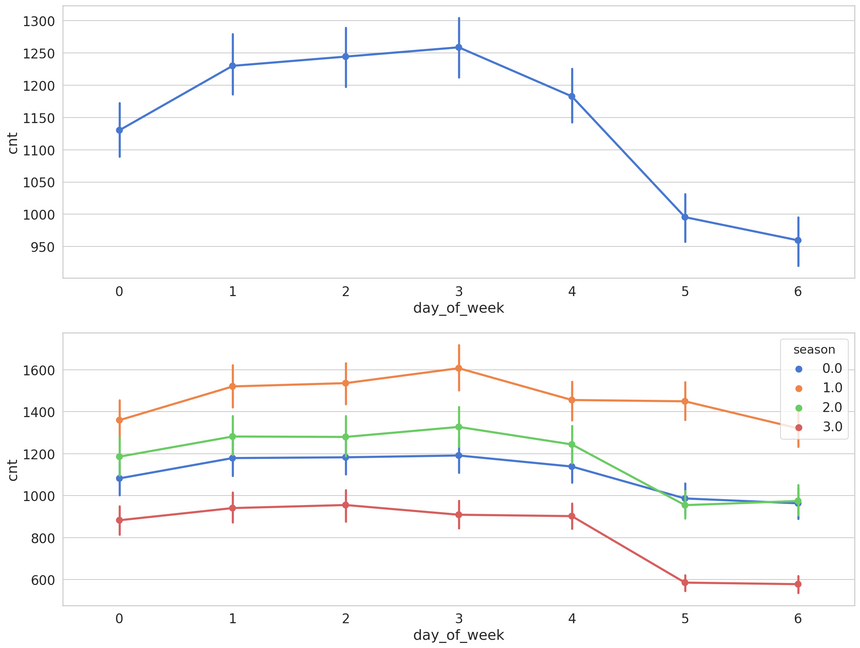Looking at the data by day of the week shows a much higher count on the number of bike shares.

Our little feature engineering efforts seem to be paying off. The new features separate the data very well.

# Preprocessing

We’ll use the last 10% of the data for testing:

1train_size = int(len(df) * 0.9)2test_size = len(df) - train_size3train, test = df.iloc[0:train_size], df.iloc[train_size:len(df)]4print(len(train), len(test))
115672 1742

We’ll scale some of the features we’re using for our modeling:

1f_columns = ['t1', 't2', 'hum', 'wind_speed']
1f_transformer = RobustScaler()23f_transformer = f_transformer.fit(train[f_columns].to_numpy())45train.loc[:, f_columns] = f_transformer.transform(6  train[f_columns].to_numpy()7)89test.loc[:, f_columns] = f_transformer.transform(10  test[f_columns].to_numpy()11)

We’ll also scale the number of bike shares too:

1cnt_transformer = RobustScaler()23cnt_transformer = cnt_transformer.fit(train[['cnt']])45train['cnt'] = cnt_transformer.transform(train[['cnt']])67test['cnt'] = cnt_transformer.transform(test[['cnt']])

To prepare the sequences, we’re going to reuse the same create_dataset() function:

1def create_dataset(X, y, time_steps=1):2    Xs, ys = [], []3    for i in range(len(X) - time_steps):4        v = X.iloc[i:(i + time_steps)].values5        Xs.append(v)6        ys.append(y.iloc[i + time_steps])7    return np.array(Xs), np.array(ys)

Each sequence is going to contain 10 data points from the history:

1time_steps = 1023# reshape to [samples, time_steps, n_features]45X_train, y_train = create_dataset(train, train.cnt, time_steps)6X_test, y_test = create_dataset(test, test.cnt, time_steps)78print(X_train.shape, y_train.shape)
1(15662, 10, 13) (15662,)

Our data is not in the correct format for training an LSTM model. How well can we predict the number of bike shares?

# Predicting Demand

Let’s start with a simple model and see how it goes. One layer of Bidirectional LSTM with a Dropout layer:

1model = keras.Sequential()2model.add(3  keras.layers.Bidirectional(4    keras.layers.LSTM(5      units=128,6      input_shape=(X_train.shape, X_train.shape)7    )8  )9)10model.add(keras.layers.Dropout(rate=0.2))11model.add(keras.layers.Dense(units=1))12model.compile(loss='mean_squared_error', optimizer='adam')

Remember to NOT shuffle the data when training:

1history = model.fit(2    X_train, y_train,3    epochs=30,4    batch_size=32,5    validation_split=0.1,6    shuffle=False7)

# Evaluation

Here’s what we have after training our model for 30 epochs: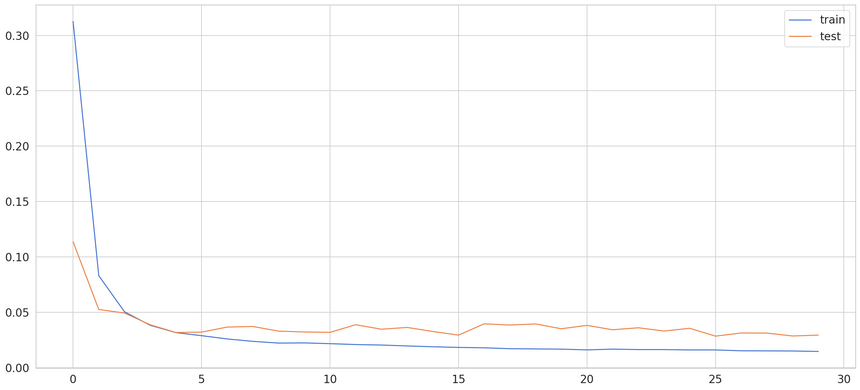You can see that the model learns pretty quickly. At about epoch 5, it is already starting to overfit a bit. You can play around - regularize it, change the number of units, etc. But how well can we predict demand with it?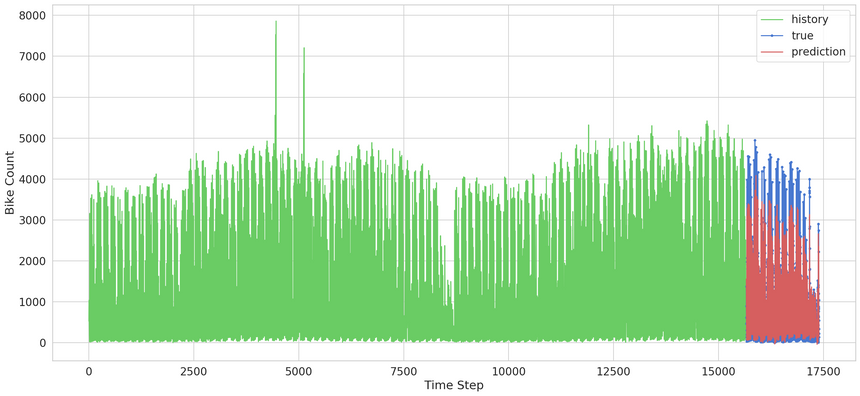That might be too much for your eyes. Let’s zoom in on the predictions: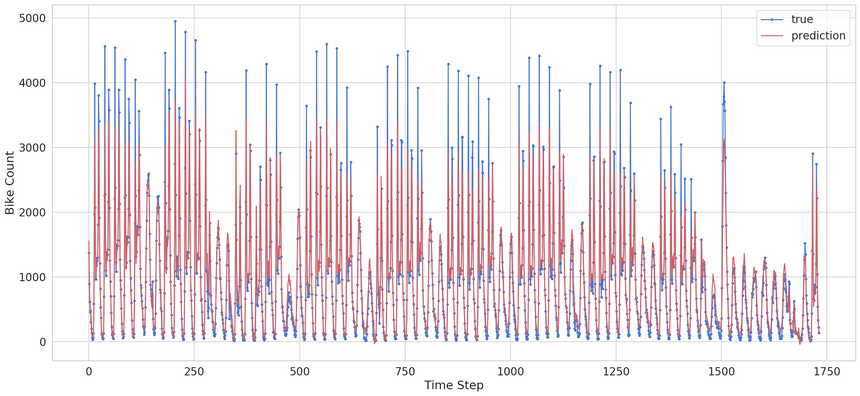Note that our model is predicting only one point in the future. That being said, it is doing very well. Although our model can’t really capture the extreme values it does a good job of predicting (understanding) the general pattern.

# Conclusion

You just took a real dataset, preprocessed it, and used it to predict bike-sharing demand. You’ve used a Bidirectional LSTM model to train it on subsequences from the original dataset. You even got some very good results.

Here are the steps you took:

Run the complete notebook in your browser

The complete project on GitHub

Are there other applications of LSTMs for Time Series data?

© 2020 Curiousily by Venelin Valkov